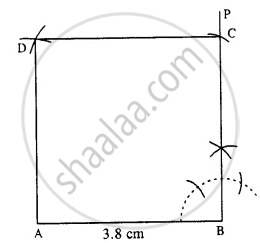# Construct a Square, If : It'S One Side is 3.8 Cm. - Mathematics

Sum

Construct a square, if :

it's one side is 3.8 cm.

#### SolutionSteps :

1. Draw AB = 3.8 cm.
2. At B, draw ∠PBA = 90°.
3. Cut BC = 3.8 cm.
4. From A and C, draw arcs of radii 3.8 cm each which intersect at D.
Thus ABCD is the required square.
Concept: Construction of Square
Is there an error in this question or solution?

#### APPEARS IN

Selina Concise Mathematics Class 8 ICSE
Chapter 18 Constructions
Exercise 18 (D) | Q 5.1 | Page 211